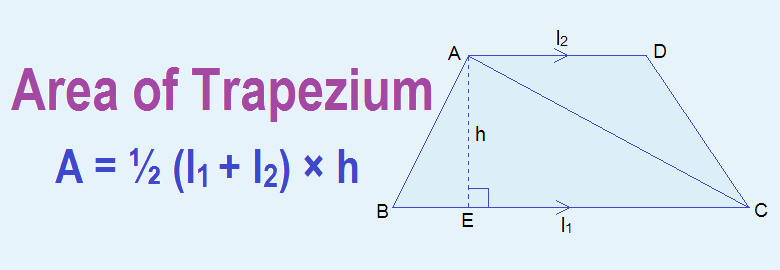# Area of Trapezium## Area of Trapezium

A trapezium is a quadrilateral having a pair of its opposite sides parallel. Area of trapezium is measured in terms of its two parallel sides and the height between them. Area of trapezium is given by the half of the product of the sum of parallel sides and height i.e. Area of trapezium = ½ (l1 + l2) × h, where l1 and l2 are two parallel sides of the trapezium and h is the height between them.

### Derivation of the Formula for Area of Trapezium

Let, ABCD in the given figure be a trapezium having AD//BC. AC is a diagonal. AEBC drawn. Diagonal AC divides the trapezium ABCD into two triangles ΔABC and ΔACD. Since perpendicular distance between two parallel lines is same everywhere, the height of both the triangles is same as the height of two parallel lines.

Let, length of two parallel lines be l1 and l2 i.e. BC = l1 AD = l2 and height between two parallel sides = AE = h

Now,
Area of trapezium ABCD = area of triangle ABC + area of triangle ACD
= ½ × BC × AE + ½ × AD × AE
= ½ (BC + AD) × AE
= ½ (l1 + l2) × h

Area of trapezium = ½ (l1 + l2) × h, where l1 and l2 are two parallel sides and h is the height between them.

### Workout Examples

Example 1: Calculate the area of given trapezium.
Solution: Here,
l1 = 9cm
l2 = 5cm
h = 4cm
Area of trapezium = ½ (l1 + l2) × h
= ½ (9cm + 5cm) × 4cm
= ½ × 14cm × 4cm
= 28cm2

Example 2: In the given figure, ABCD is a trapezium in which AD//BC, ABC = 90°, AB = 15cm and DC = 17cm. If the area of trapezium ABCD is 204cm2, calculate the length of AD.
Solution: DEBC drawn,
From the figure,
AB = DE = 15cm, DC = 17cm
In right angle ΔDEC,

Now,
Area of trapezium ABCD = 204 cm2
i.e.          ½ (AD + BC) × AB = 204
or,          ½ (AD + BE + EC) × 15 = 204